Vector field on a manifoldA section of the tangent bundle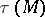. The set of differentiable vector fields forms a module over the ringof differentiable functions on.

Contents

Example 1.

For a chart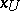of the manifoldone defines the-th basic vector field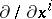according to the formulawhere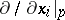is the-th basic tangent vector toat the point. Any vector fieldcan be uniquely represented in the form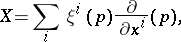where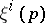are the components ofin. Since a vector field can be regarded as a derivation of the ring(see example 2), the set of vector fields forms a Lie algebra with respect to the commutation operation (the Lie bracket).

Example 2.

For the chartand, the functionis defined by the formula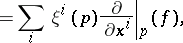whereis the partial derivative with respect to. Note that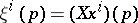;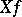is called the derivative ofin the direction.

Example 3.

For the chartand, the commutator (Lie bracket)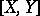of the vector fieldsis defined by the formula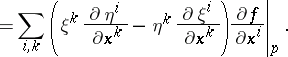It satisfies the relations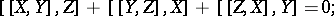in particular,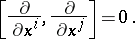Each vector fieldinduces a local flow on— a family of diffeomorphisms of a neighbourhood,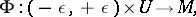such that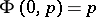for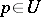and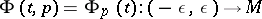is the integral curve of the vector fieldthrough, i.e.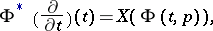where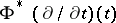is the tangent vector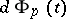toat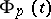. Conversely, a vector fieldis associated with a local flow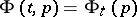, which is a variation of the mapping; hereEach vector field defines a Lie derivation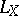of a tensor field of typewith values in a vector space (infinitesimal transformation of), corresponding to the local flow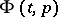; its special cases include the action of the vector field on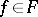,and the Lie bracket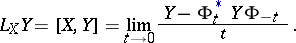A vector field without singularities generates an integrable one-dimensional differential system as well as a Pfaffian system associated with it on.

A generalization of the concept of a vector field on a manifold is that of a vector field along a mapping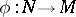, which is a section of the bundle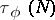induced by, as well as a tensor field of type, which is a section of the bundleassociated withwith the aid of the functor.